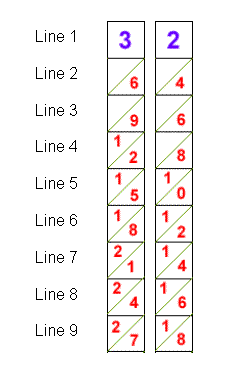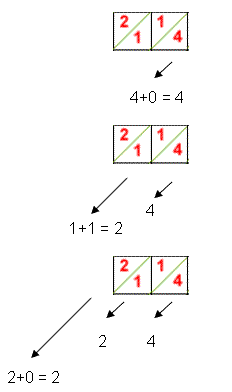#### You may also like### Prompt Cards

These two group activities use mathematical reasoning - one is numerical, one geometric.### Consecutive Numbers

An investigation involving adding and subtracting sets of consecutive numbers. Lots to find out, lots to explore.### Exploring Wild & Wonderful Number Patterns

EWWNP means Exploring Wild and Wonderful Number Patterns Created by Yourself! Investigate what happens if we create number patterns using some simple rules.

# Napier's Bones

##### Age 7 to 11 Challenge Level:

The table is split up into the nine columns. Here is how they're used:

For example, let's calculate 32 x 7 .

Lay out the two strips showing 3 and 2 at the top like this:To multiply this number by 7, add the numbers along the diagonals of the seventh line, starting at the right:So the answer is 224 .

Here's another example, this time we are multiplying 746 by 6. Click on Napier to start the demo.